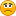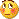# Std Deviation - page 24

Is There a Hi vs Low Over Time Indicator?

Hi everyone! I'm new here (and relatively new to Forex tading).

I was wondering if there is an indicator that plots a line representing the DIFFERENCE between the highest close and the lowest close over a user-defined period of time. It's a kind of a volatility thing, but different from what I've seen. What I'd like to be able to do is look at this indicator and know whether the price is (for example) even covering the spread at a particular point in time. So if the spread was 3 pips, and the indicator told me that over the last 14 periods (or whatever number of period I set it to) the highest close and the lowest close have ony been 2.5 pips apart, there's little point in trying to enter beacuse the avarage change over this period of time hasn't been enough to even cover the spread, let alon some higher profit point.

Am I making myself clear on this? If not, I can try again. But is there is such an indicator and if so, what/where is it? And if not, could any of you programming types make one? :-)

Thanks much!!

Scott <

_______________________________________

Scott Sutherland

484 Yorkshire Dr.

Oviedo, FL 32765

ScottPSutherland@gmail.com11

Standard Deviation of an Indicator

1) find standard deviation of the indicator (whichever indicator I drag this into) and use the last standard deviation value

2) draw 2 lines above zero and two lines below zero such that:

first line above zero (green) is 1 standard deviation

second line above zero (red) is 2 standard deviations

first line below zero (green) is -1 standard deviation

second line below zero (red) is -2 standard deviations

thanks..154334

...

Was looking for some calculations and when I did not find those for metatrader...

Now, this is made as an indicator but it is rather a tool for calculating certain values for arrays than an indicator

I could not think of a better name for it than deviations (based on the majority of work it does:))

______________________

Parameters are simple except maybe for the modeparameter

Modeparameter values :
0 - A simple moving average (nothing else)

1 - variance (Variance - Wikipedia, the free encyclopedia)

2 - average (absolute) deviation (https://en.wikipedia.org/wiki/Absolute_deviation

3 - standard deviation (Standard deviation - Wikipedia, the free encyclopedia)

4 - skewness (Skewness - Wikipedia, the free encyclopedia)

5 - kurtosis (Kurtosis - Wikipedia, the free encyclopedia)

______________________

As I told, as I could not think of a better name for this and also I did not find a better thread to post it to.

Files:
devs.gif 26 kb11

standard deviation of an indicator

As I tried to explain above, I need the last standard deviation value of the indicator used. This value is a number that takes all history of the indicator into consideration. The reason I say last standard deviation value is that, you will have a standard deviation value for each data point that used the data up to this point, however what I need is using the whole history.

After finding the standard deviation, the rest is simpler.

Just multiply it by +1,+2,-1 and -2 and draw it (preferably with +1/-1 in green, +2/-2 in red).

The reason for using this: I'm using indicators that does not have definite values (eg. unlike RSI 0-100 with 30-70 lines or stochastic 0-100 with 20-80 lines). Using the standard deviation lines, we can find the historical extreme values of these indicators and be warned when they are overextended.

I don't have any experience with MT4 language, please help me with this relatively easy script. If there is no formula for standard deviation inside the MT4 editor, I can provide the formula for it as well.

Thanks..

Files:154334

In the deviations indicator above your post you have a line (line 134) that goes like this :

prices = iMA(NULL,0,1,0,MODE_SMA,price,i);[/php]replace the iMA(...) part (which is, in deviations indicator used only to extract prices faster) with whatever indicator you want to calculate standard deviation for and set mode to 3 (for example :

[php] prices = iRSI(NULL,0,RsiPeriod,RsiPrice,i);

buffer = iCalc(prices,StdDevPeriod,3,i);

would calculate standard deviation of rsi for RsiPeriod, RsiPrice and StdDevPeriod (which you have to provide))

________________________

You can also use the iStdDevOnArray() function provided that you fill some array similarly like from the example above...

mzeki:
As I tried to explain above, I need the last standard deviation value of the indicator used. This value is a number that takes all history of the indicator into consideration. The reason I say last standard deviation value is that, you will have a standard deviation value for each data point that used the data up to this point, however what I need is using the whole history.

After finding the standard deviation, the rest is simpler.

Just multiply it by +1,+2,-1 and -2 and draw it (preferably with +1/-1 in green, +2/-2 in red).

The reason for using this: I'm using indicators that does not have definite values (eg. unlike RSI 0-100 with 30-70 lines or stochastic 0-100 with 20-80 lines). Using the standard deviation lines, we can find the historical extreme values of these indicators and be warned when they are overextended.

I don't have any experience with MT4 language, please help me with this relatively easy script. If there is no formula for standard deviation inside the MT4 editor, I can provide the formula for it as well.

Thanks..339

Was looking for some calculations and when I did not find those for metatrader...

Now, this is made as an indicator but it is rather a tool for calculating certain values for arrays than an indicator

I could not think of a better name for it than deviations (based on the majority of work it does:))

______________________

Parameters are simple except maybe for the modeparameter

Modeparameter values :
0 - A simple moving average (nothing else)

1 - variance (Variance - Wikipedia, the free encyclopedia)

2 - average (absolute) deviation (https://en.wikipedia.org/wiki/Absolute_deviation

3 - standard deviation (Standard deviation - Wikipedia, the free encyclopedia)

4 - skewness (Skewness - Wikipedia, the free encyclopedia)

5 - kurtosis (Kurtosis - Wikipedia, the free encyclopedia)

______________________ As I told, as I could not think of a better name for this and also I did not find a better thread to post it to.

Superb, thank you.9493

Was looking for some calculations and when I did not find those for metatrader...

Now, this is made as an indicator but it is rather a tool for calculating certain values for arrays than an indicator

I could not think of a better name for it than deviations (based on the majority of work it does)

______________________

Parameters are simple except maybe for the mode parameter

Mode parameter values :

0 - A simple moving average (nothing else )

1 - variance (Variance - Wikipedia, the free encyclopedia)

2 - average (absolute) deviation (Absolute deviation - Wikipedia, the free encyclopedia)

3 - standard deviation (Standard deviation - Wikipedia, the free encyclopedia)

4 - skewness (Skewness - Wikipedia, the free encyclopedia)

5 - kurtosis (Kurtosis - Wikipedia, the free encyclopedia)

______________________

As I told, as I could not think of a better name for this and also I did not find a better thread to post it to.

Thank you Mladen,here's a picture of AllMa ZeroLag using kurtosis, verry,verry interesting!!!!!11

Standard Deviation of an Indicator

I tried what you said (really tried hard) but again failedCan you please post here the full code with changes.

Instead of mentioning any indicator (like RSI) inside the code, isn't it possible to use it for any indicator that the new indicator is attached to, just like Moving Average of Oscillator..9493

Deviations

Here's RSI Deviation and ATR Deviation196

Thanks MT

Thanks for sharing Mr Tools. I am looking forward to watching these this week.